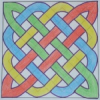Search by Topic

Filter by: Content type:
Age range:
Challenge level:

There are 22 NRICH Mathematical resources connected to Common factors, you may find related items under Numbers and the Number System.

Broad Topics > Numbers and the Number System > Common factorsCounting Cogs

Age 7 to 14 Challenge Level:

Which pairs of cogs let the coloured tooth touch every tooth on the other cog? Which pairs do not let this happen? Why?What's in the Box?

Age 7 to 11 Challenge Level:

This big box multiplies anything that goes inside it by the same number. If you know the numbers that come out, what multiplication might be going on in the box?Clapping Times

Age 5 to 7 Challenge Level:

If you count from 1 to 20 and clap more loudly on the numbers in the two times table, as well as saying those numbers loudly, which numbers will be loud?Stars

Age 11 to 14 Challenge Level:

Can you find a relationship between the number of dots on the circle and the number of steps that will ensure that all points are hit?Multiplication Squares

Age 7 to 11 Challenge Level:

Can you work out the arrangement of the digits in the square so that the given products are correct? The numbers 1 - 9 may be used once and once only.Common Divisor

Age 14 to 16 Challenge Level:

Find the largest integer which divides every member of the following sequence: 1^5-1, 2^5-2, 3^5-3, ... n^5-n.Em'power'ed

Age 16 to 18 Challenge Level:

Find the smallest numbers a, b, and c such that: a^2 = 2b^3 = 3c^5 What can you say about other solutions to this problem?Biscuit Decorations

Age 5 to 7 Challenge Level:

Andrew decorated 20 biscuits to take to a party. He lined them up and put icing on every second biscuit and different decorations on other biscuits. How many biscuits weren't decorated?Sieve of Eratosthenes

Age 11 to 14 Challenge Level:

Follow this recipe for sieving numbers and see what interesting patterns emerge.Missing Multipliers

Age 7 to 14 Challenge Level:

What is the smallest number of answers you need to reveal in order to work out the missing headers?Drawing Celtic Knots

Age 11 to 14 Challenge Level:

Here is a chance to create some Celtic knots and explore the mathematics behind them.A First Product Sudoku

Age 11 to 14 Challenge Level:

Given the products of adjacent cells, can you complete this Sudoku?There's Always One Isn't There

Age 14 to 16 Challenge Level:

Take any pair of numbers, say 9 and 14. Take the larger number, fourteen, and count up in 14s. Then divide each of those values by the 9, and look at the remainders.Turning Cogs

Age 7 to 11 Challenge Level:

What happens when you turn these cogs? Investigate the differences between turning two cogs of different sizes and two cogs which are the same.Data Chunks

Age 14 to 16 Challenge Level:

Data is sent in chunks of two different sizes - a yellow chunk has 5 characters and a blue chunk has 9 characters. A data slot of size 31 cannot be exactly filled with a combination of yellow and. . . .Different by One

Age 14 to 16 Challenge Level:

Make a line of green and a line of yellow rods so that the lines differ in length by one (a white rod)Spelling Circle

Age 7 to 11 Challenge Level:

Find the words hidden inside each of the circles by counting around a certain number of spaces to find each letter in turn.Cogs

Age 11 to 14 Challenge Level:

A and B are two interlocking cogwheels having p teeth and q teeth respectively. One tooth on B is painted red. Find the values of p and q for which the red tooth on B contacts every gap on the. . . .Inclusion Exclusion

Age 11 to 14 Challenge Level:

How many integers between 1 and 1200 are NOT multiples of any of the numbers 2, 3 or 5?Ewa's Eggs

Age 11 to 14 Challenge Level:

I put eggs into a basket in groups of 7 and noticed that I could easily have divided them into piles of 2, 3, 4, 5 or 6 and always have one left over. How many eggs were in the basket?Hypotenuse Lattice Points

Age 14 to 16 Challenge Level:

The triangle OMN has vertices on the axes with whole number co-ordinates. How many points with whole number coordinates are there on the hypotenuse MN?DOTS Division

Age 14 to 16 Challenge Level:

Take any pair of two digit numbers x=ab and y=cd where, without loss of generality, ab > cd . Form two 4 digit numbers r=abcd and s=cdab and calculate: {r^2 - s^2} /{x^2 - y^2}.